﻿

# 基于CO2排放量数据集的数据分析及可视化

【版权声明】版权所有，严禁转载，严禁用于商业用途，侵权必究。

# 一、实验环境

Linux：Ubuntu18.04
Spark：3.2.0
Python3.7
Scala：2.12.15
Jupyter notebook

# 二、数据预处理

## 2.1 数据集简介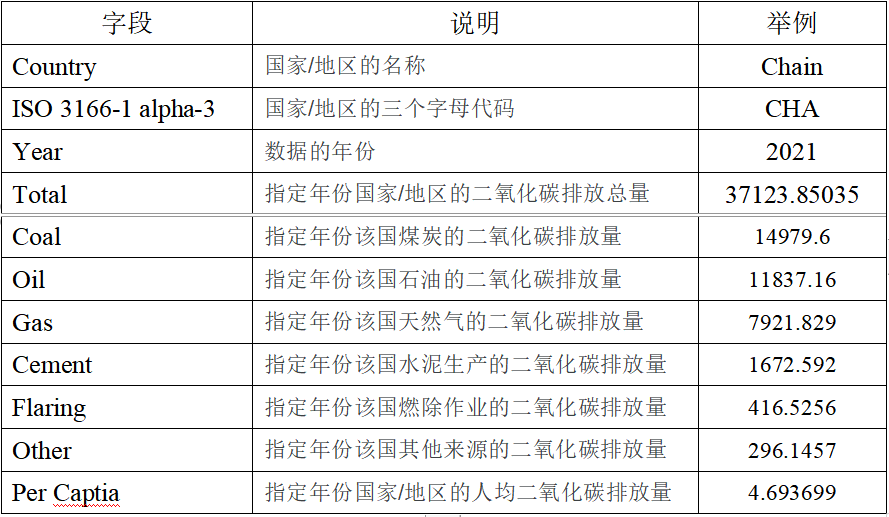## 2.2 数据集清洗

1.首先国家的ISO 3166-1 alpha-3代码对本文的分析没有多大用处，就过滤掉该字段。
2.由于该数据是从1750年开始收集的，由于种种原因，总有一些国家的某些年数据是丢失的。因此，过滤掉某个国家某年数据全为空的行。
3.该数据集中存在“global 和 International Transport”。这两个字段表示全球和国际排放量。特别地，这两个数据不代表国家，因此过滤掉这两个数据。

``````1.# 数据处理文件，处理数据集
2.import pandas as pd
5.# 去除字段国家简称字段
6.# 过滤掉total = 0的字段,填充nan到0
7.# 去除global 和 International Transport的数据
8.data = data.fillna(0)
9.with open('dataset.csv','w+',encoding='utf-8') as f:
10.    for line in data.values:
11.        if line == 0.0:
12.            continue
13.        if line == "Global" or line == "International Transport":
14.            continue
15.        f.write(str(line)+'\t'+str(line)+'\t'
16.                +str(line)+'\t'+str(line)+'\t'+str(line)+'\t'+
17.                str(line)+'\t'+str(line)+'\t'+str(line)+'\t'+
18.                str(line)+'\t'+str(line)+'\n')  ``````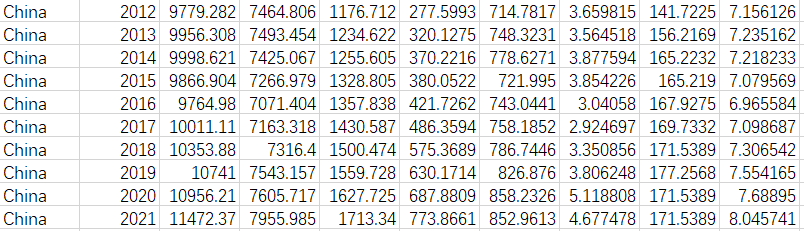## 2.3 启动HDFS和Spark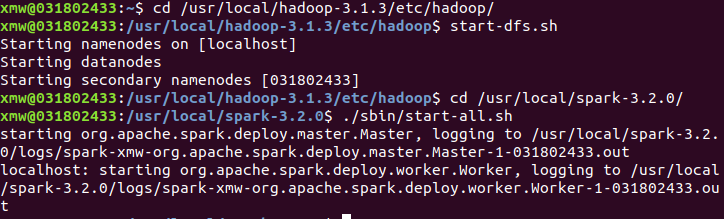## 2.4 上传数据到HDFS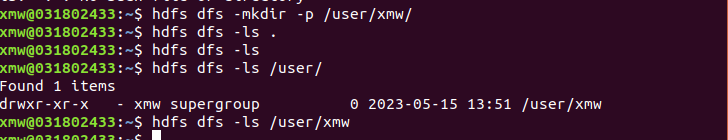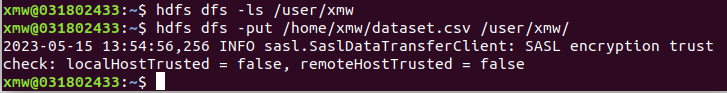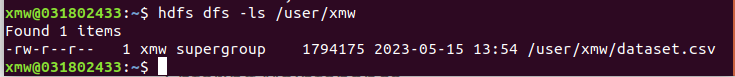# 三、使用 Spark 将数据转为 DataFrame

``````1.import findspark
2.findspark.init()
3.from pyspark import SparkContext
4.from pyspark.sql import SparkSession, Row
5.from pyspark.sql.functions import col, max
6.from pyspark.sql.types import StringType, StructField, StructType,IntegerType,FloatType
7.import json
8.import csv
9.import os
10.from pyspark.sql import functions as F
11.sc = SparkContext('local', 'spark_project')
12.sc.setLogLevel('WARN')
13.spark = SparkSession.builder.getOrCreate()
14.
15.schemaString = "Country,Year,Total,Coal,Oil,Gas,Cement,Flaring,Other,PerCapita"
16.fields = []
17.for field in schemaString.split(","):
18.    if field == 'Country':
19.        a = StructField(field, StringType(), True)
20.    elif field == 'Year':
21.        a = StructField(field,IntegerType(),True)
22.    else:
23.        a = StructField(field,FloatType(),True)
24.    fields.append(a)
25.# fields.append(a)
26.schema = StructType(fields)
27.CO2Rdd1 = sc.textFile('/user/xmw/dataset.csv')
28.CO2Rdd = CO2Rdd1.map(lambda x:x.split("\t")).map(lambda p: Row(p,int(p),float(p),float(p),float(p),float(p),float(p),float(p),float(p),float(p)))
29.mdf = spark.createDataFrame(CO2Rdd, schema)
30.mdf.createOrReplaceTempView("usInfo")
31.mdf.show()
32.# 展示数据表结构
33.mdf.printSchema()  ``````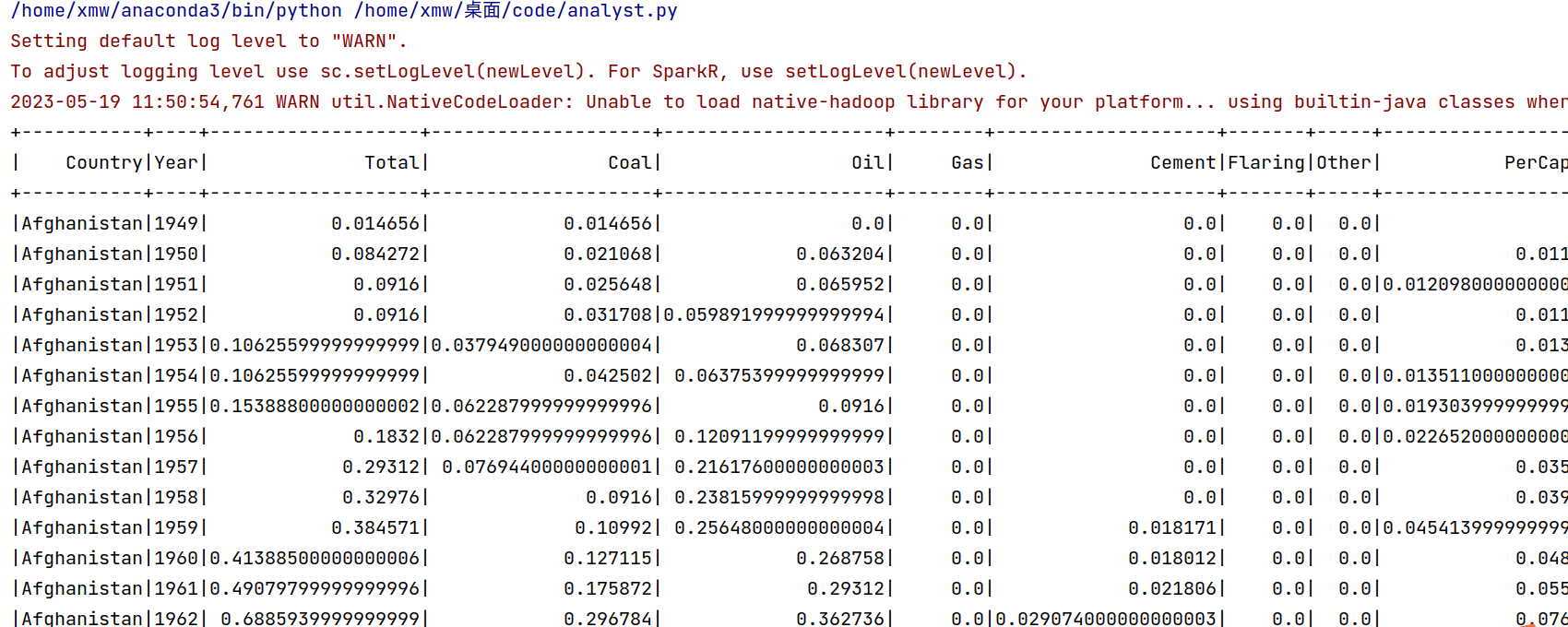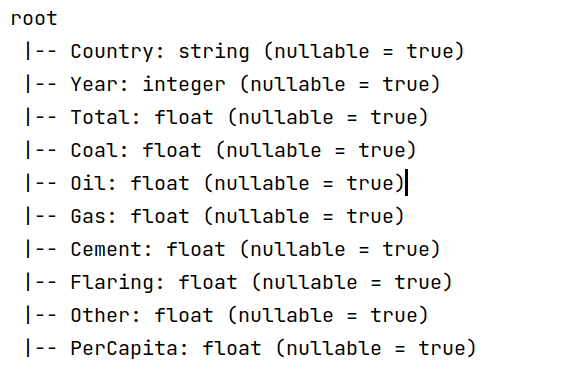# 四、使用 Spark 对 HDFS 中的数据进行分析

## 1. 查询2021年中国的排放数据

``````1.# 1.查询2021年中国的排放总量
2.df1 = spark.sql(
3.    "SELECT * FROM usInfo WHERE Country = 'China' AND Year = '2021'")
4.# 将结果保存为文本文件
5.df1.repartition(1).write.csv('/user/xmw/df1',mode="overwrite")
6.df1.show()  ``````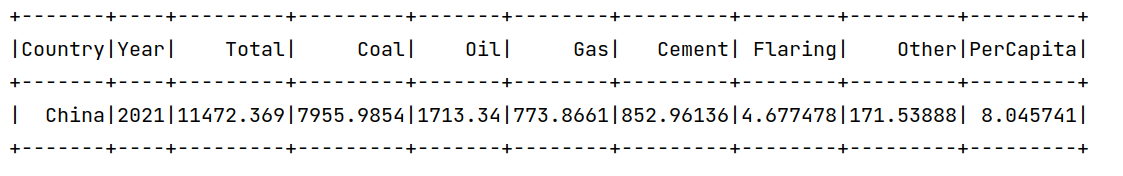## 2. 统计总CO2排放量

``````1.# 2.统计总能源使用量
2.df2 = mdf.selectExpr("SUM(Total) AS total_energy")
3.df2.show()
4.df2.repartition(1).write.csv('/user/xmw/df2',mode="overwrite")  ``````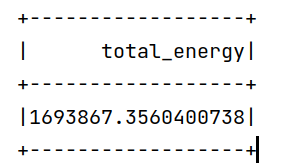## 3. 计算每个国家的平均人均能源使用量

``````1.# 3.计算每个国家的平均人均能源使用量(总的)，不是每一年的
2.df3 = mdf.groupBy("Country").agg({"PerCapita": "avg"}).withColumnRenamed("avg(PerCapita)", "AvgPerCapita")
3.df3.show()
4.df3.repartition(1).write.csv('/user/xmw/df3',mode="overwrite")  ``````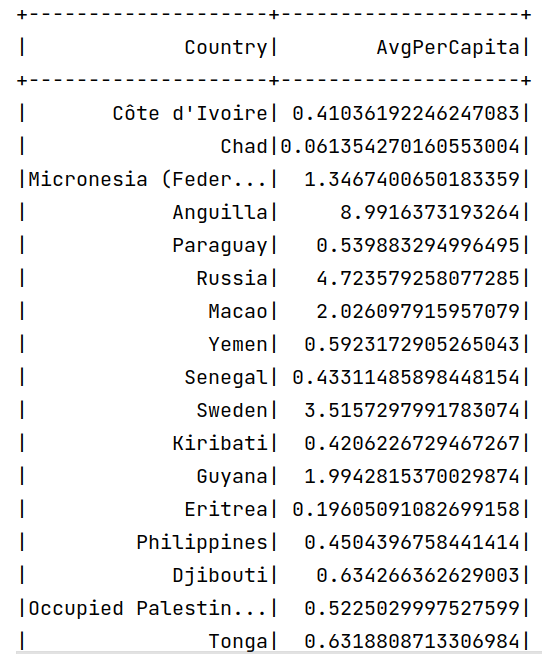## 4. 获取排放总量最高的国家和对应的年份

``````1.#4.获取排放总量最高的国家和对应的年份
2.df4 = mdf.select("Country", "Year", "Total").orderBy(F.col("Total").desc())
3.df4.show(1)
4.df4.repartition(1).write.csv('/user/xmw/df4',mode="overwrite")
5.print("排放总量最高的国家:", df4.first()["Country"])
6.print("对应的年份:", df4.first()["Year"])  ``````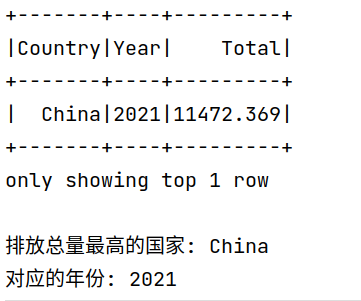## 5. 2021年人均排放最高的国家

``````1.#5.2021年人均排放最高的国家
2.df_2021 = mdf.filter(mdf.Year == 2021)
3.# 按人均排放降序排序并获取第一条记录
4.df5 = df_2021.orderBy(F.col("PerCapita").desc())
5.df5.show(1)
6.df5.repartition(1).write.csv('/user/xmw/df5',mode="overwrite")
7.# 输出人均排放最高的国家和对应的人均排放值
8.country = df5.first()["Country"]
9.per_capita = df5.first()["PerCapita"]
10.print("2021年人均排放最高的国家:", country)
11.print("人均排放值:", per_capita)  ``````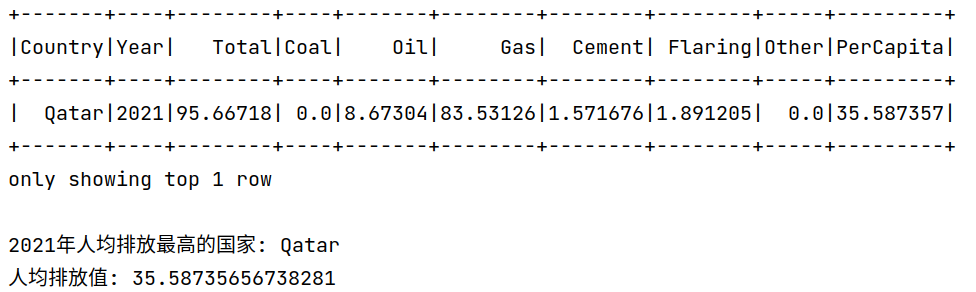## 6. 中国历史排放总量（2000年后）

``````1.#6. 中国历史排放总量（2000-2021）
2.# 过滤出中国2000年以后的数据
3.df6 = mdf.filter((mdf.Country == "China") & (mdf.Year >= 2000))
4.df6.show()
5.df6.repartition(1).write.csv('/user/xmw/df6',mode="overwrite")  ``````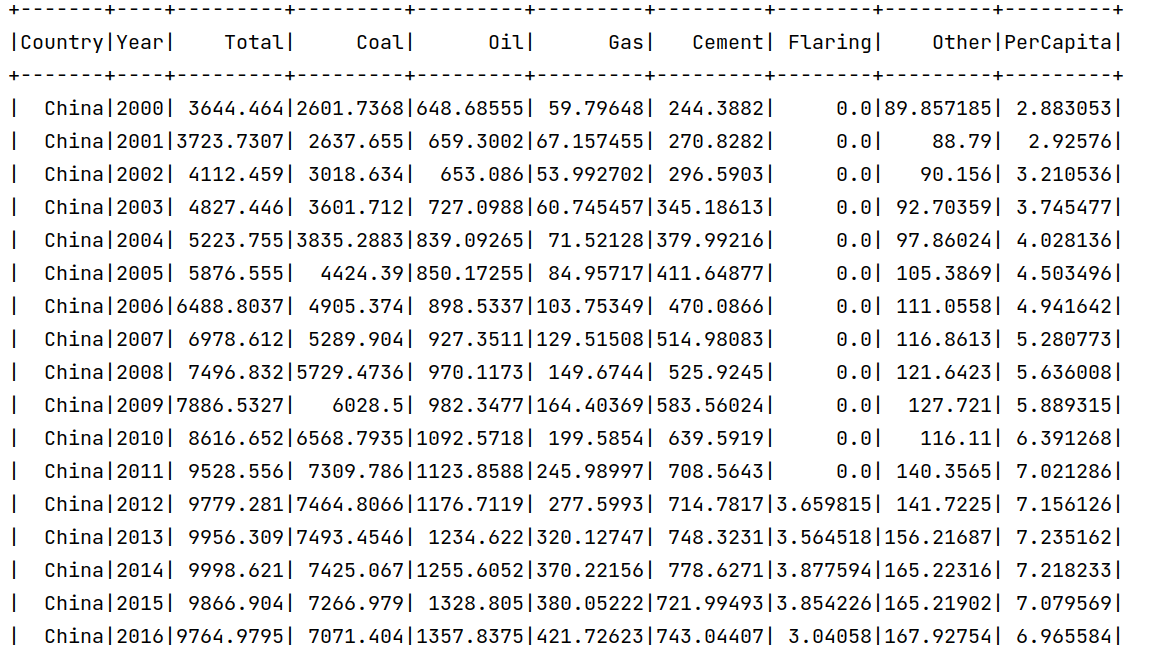## 7. 查询每年排放总量最高的国家

``````1.7. 查询每年排放总量最高的国家
2.使用 Spark SQL 查询每年排放总量最高的国家
3.query = """
4.    SELECT Year, Country, Total
5.    FROM (
6.        SELECT Year, Country, Total, ROW_NUMBER() OVER (PARTITION BY Year ORDER BY Total DESC) as rank
7.        FROM usInfo
8.    ) ranked
9.    WHERE rank = 1
10.    ORDER BY Year
11."""
12.df7 = spark.sql(query)
13.df7.repartition(1).write.csv('/user/xmw/df7',mode="overwrite")
14.# 显示所有查询结果
15.df7.show(300) ``````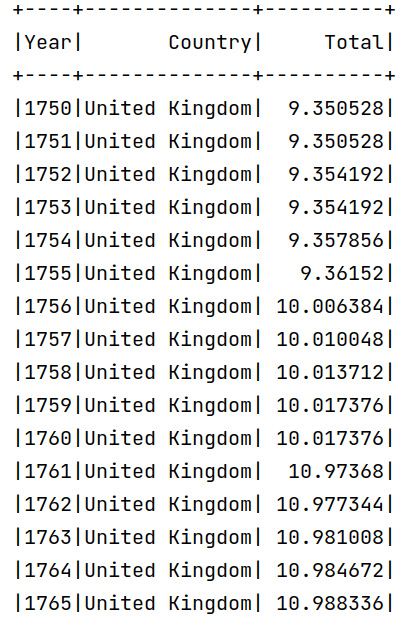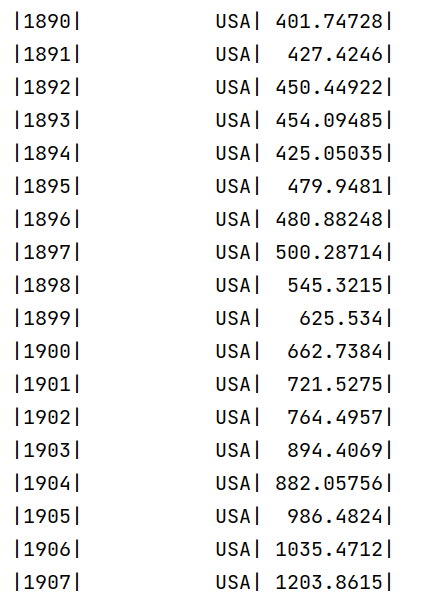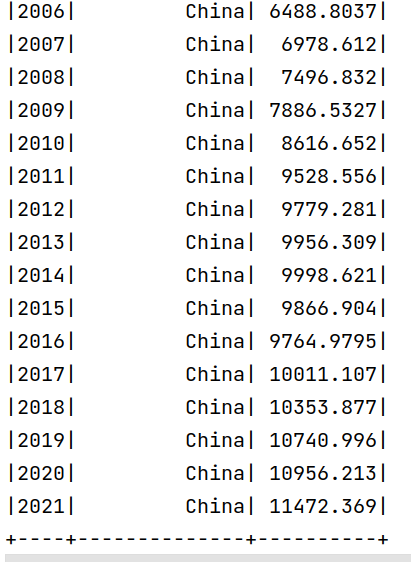## 8. 2021年排放前10的国家

``````1.# 8.2021年排放前10的国家
2.# 过滤出2021年的数据
3.df_2021 = mdf.filter(mdf.Year == "2021")
4.# 按排放总量降序排序，并选取前10名国家
5.df8 = df_2021.orderBy(df_2021.Total.desc()).limit(10)
6.df8.repartition(1).write.csv('/user/xmw/df8',mode="overwrite")
7.# 显示查询结果
8.df8.show() ``````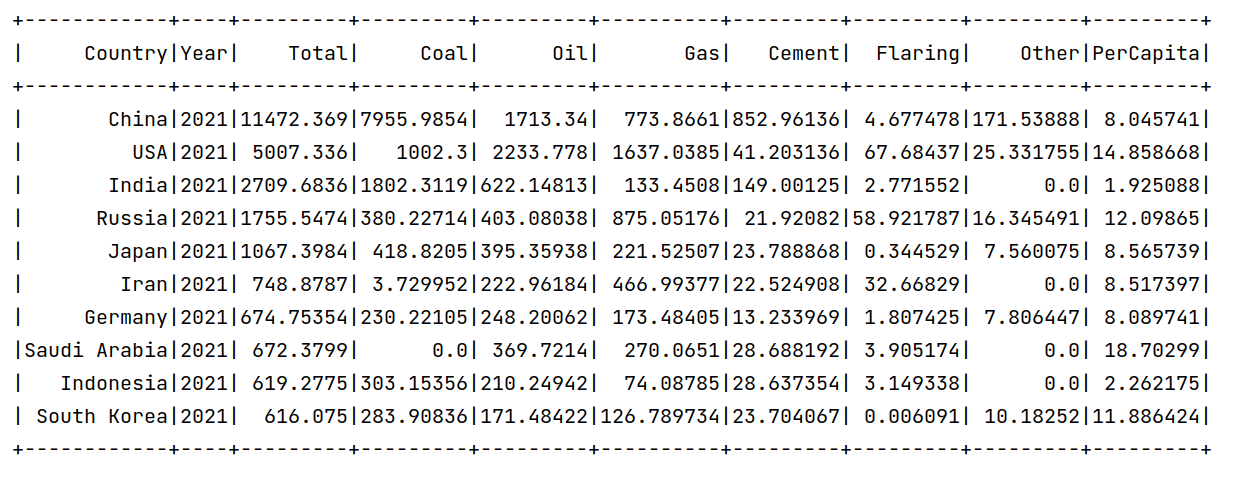## 9. 每个国家的历史排放总量之和，并且按照从高到低排序

``````1.# 9. 每个国家的历史排放总量之和，并且按照从高到低排序
2.query = """
3.    SELECT Country, SUM(Total) AS TotalSum
4.    FROM usInfo
5.    GROUP BY Country
6.    ORDER BY TotalSum DESC
7."""
8.df9 = spark.sql(query)
9.# 显示查询结果
10.df9.show()
11.df9.repartition(1).write.csv('/user/xmw/df9',mode="overwrite")  ``````

## 10．计算每个国家的排放总量占比

``````1.# 10.   计算每个国家的排放总量占比
2.# 计算全局的排放总量
3.global_total = spark.sql("SELECT SUM(Total) FROM usInfo").collect()
4.# 使用 Spark SQL 查询每个国家的排放总量及占比
5.query = f"""
6.    SELECT Country, SUM(Total) AS TotalEmissions, SUM(Total) / {global_total} * 100 AS Percentage
7.    FROM usInfo
8.    GROUP BY Country
9.    ORDER BY TotalEmissions DESC
10."""
11.df10 = spark.sql(query)
12.df10.repartition(1).write.csv('/user/xmw/df10',mode="overwrite")
13.# 显示查询结果
14.df10.show()  ``````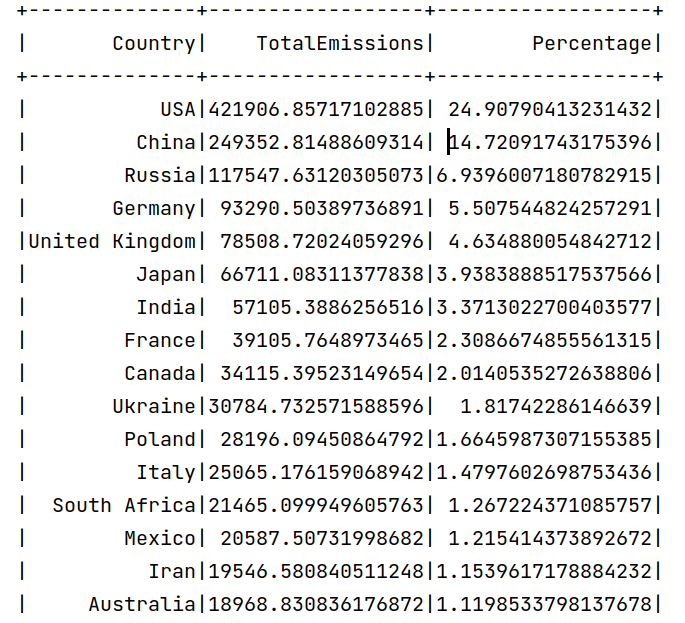## 11. 计算煤炭、石油、天然气、水泥、燃烧和其他排放总量占比

``````1.# 11.查询语句改写计算煤、油、气、水泥、燃烧和其他排放总量占比
2.# 使用 Spark SQL 计算每个字段的总量
3.query = """
4.    SELECT
5.        SUM(Coal) AS CoalTotal,
6.        SUM(Oil) AS OilTotal,
7.        SUM(Gas) AS GasTotal,
8.        SUM(Cement) AS CementTotal,
9.        SUM(Flaring) AS FlaringTotal,
10.        SUM(Other) AS OtherTotal
11.    FROM usInfo
12."""
13.df11 = spark.sql(query)
14.df11.repartition(1).write.csv('/user/xmw/df11',mode="overwrite")
15.df11 = df11.collect()
16.# 计算总排放量
17.total = sum(df11)
18.
19.# 计算每个字段的占比
20.emission_percentages = {
21.    field: (df11[field] / total) * 100
22.    for field in df11.asDict().keys()
23.}
24.# 打印每个字段的占比
25.for field, percentage in emission_percentages.items():
26.    print(f"{field}: {percentage:.2f}%")  ``````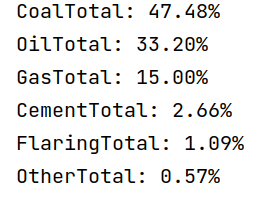# 五、使用 Spark MLlib 组件进行数据分析

## 1. 相关性分析

``````1.# 将需要进行相关性分析的字段进行向量化
2.assembler = VectorAssembler(
3.    inputCols=["Year", "Total", "Coal", "Oil", "Gas", "Cement", "Flaring", "Other", "PerCapita"],
4.    outputCol="features"
5.)
6.df = assembler.transform(mdf).select("features")
7.# 计算字段之间的相关系数
9.# 提取相关系数矩阵
10.correlation_matrix_array = correlation_matrix.toArray()
11.# 打印相关系数矩阵
12.print(correlation_matrix_array)
13.# 创建 DataFrame，保存到 HDFS
14.result_df = spark.createDataFrame(correlation_matrix_array.tolist())
15.result_df.write.mode("overwrite").format("csv").save("/user/xmw/analyst-ml-1")  ``````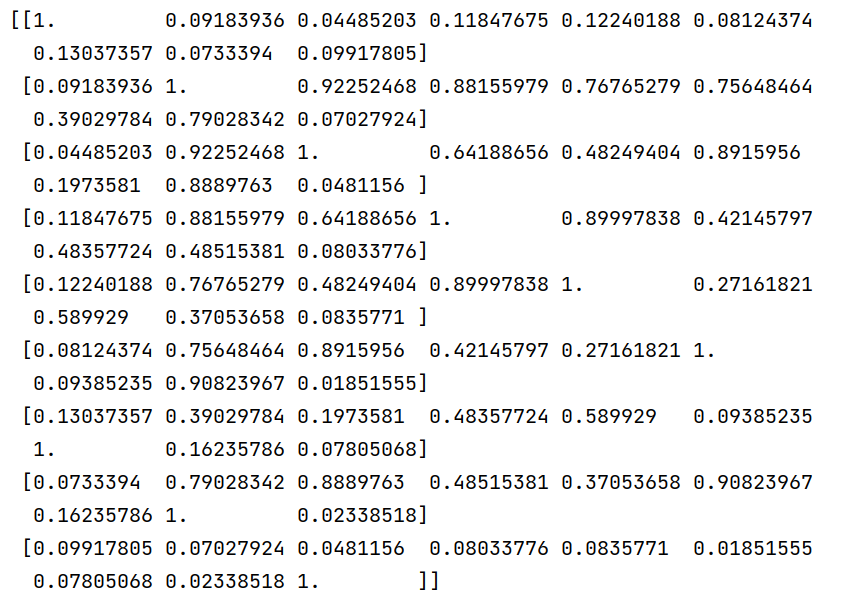## 2.使用回归分析，预测中国2023年的总CO2排放量

``````1.# 筛选特定国家的数据
2.country = "China"  # 替换为你要预测的国家名称
3.country_df = mdf.filter(mdf.Country == country)
4.# 将字段进行向量化
5.assembler = VectorAssembler(
6.    inputCols=["Year"],
7.    outputCol="features"
8.)
9.country_df = assembler.transform(country_df)
10.# 创建 LinearRegression 模型
11.lr = LinearRegression(featuresCol="features", labelCol="Total")
12.# 拟合模型
13.model = lr.fit(country_df)
14.# 构造要预测的数据
15.prediction_data = spark.createDataFrame([(2023,)], ["Year"])
16.# 将要预测的数据进行特征向量化
17.prediction_data = assembler.transform(prediction_data)
18.# 进行预测
19.predictions = model.transform(prediction_data)
20.# 提取预测结果
21.predicted_total = predictions.select("prediction").collect()
22.# 打印预测结果
23.print(f"Predicted Total Emission for {country} in 2023: {predicted_total}")
24.# 保存预测结果到 HDFS
25.result_df = spark.createDataFrame([(country,2023, predicted_total)], ["Country", "Year","PredictedTotal"])
26.result_df.write.mode("overwrite").format("csv").save("/user/xmw/analyst-ml-2")  ``````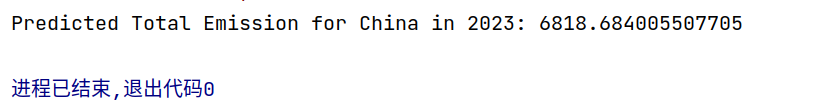## 3. 使用 Summarizer 函数统计信息

``````1.# 特征选择和转换
2.assembler = VectorAssembler(
3.    inputCols=["Coal", "Oil", "Gas", "Cement", "Flaring", "Other"],
4.    outputCol="features"
5.)
6.df = assembler.transform(mdf)
7.# 计算汇总统计信息
8.summary = Summarizer.metrics("mean", "max", "min", "std")
9.# 应用 Summarizer 并计算摘要统计信息
10.summary_df = df.select(summary.summary(df.features).alias("summary"))
11.# 显示摘要统计信息
12.summary_df.show(truncate=False)
13.# 将结果保存为 Parquet 文件
14.summary_df.write.parquet("/user/xmw/analyst-ml-3")  ``````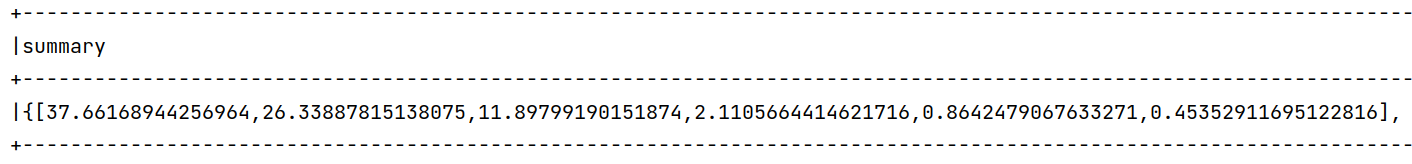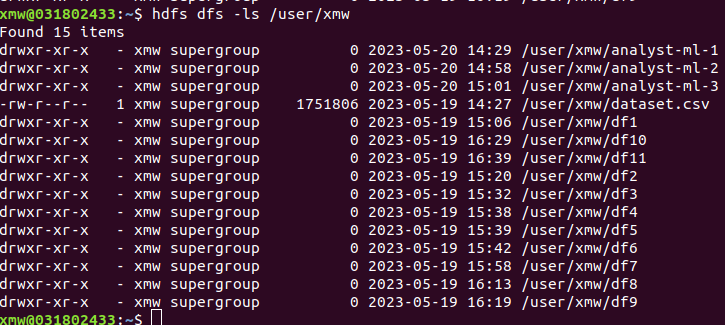# 六、数据可视化

## 1.总排放量前10的国家

``````1.# 1. 排放量前10的国家
2.countries = list(df.groupby('Country').sum().sort_values(by='Total',ascending=False).index)
3.values = list(df.groupby('Country').sum().sort_values(by='Total',ascending=False)['Total'])
4.
5.plt.figure(figsize=(12,5))
6.sns.set_style('darkgrid')
7.sns.barplot(x=countries[:10],y=values[:10],palette='Set2',edgecolor='.2')  ``````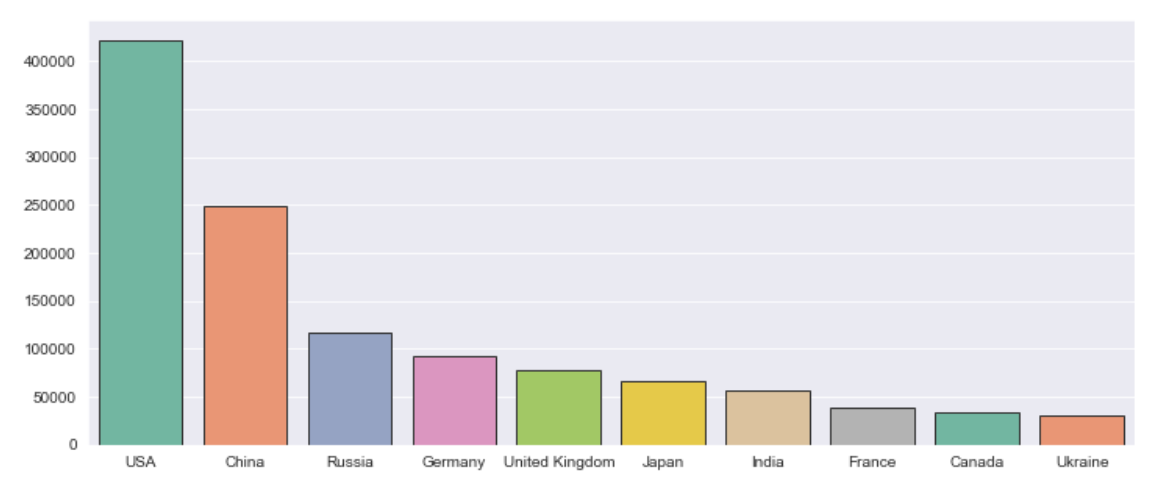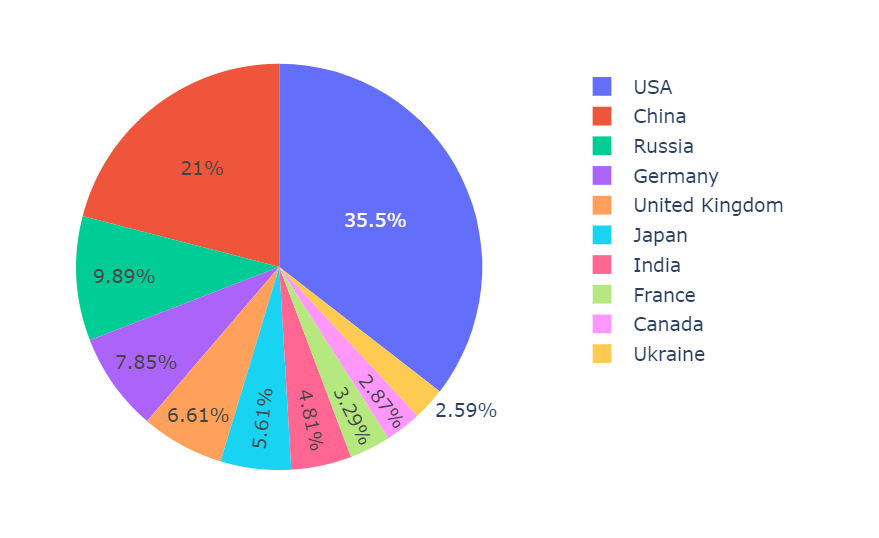## 2.中国在2012-2021的总排放量趋势

``````1.# 2.中国相关数据可视化
2.china_data = df[df['Country']=='China']
3.# 前10年的变化
4.china_past_10 = china_data[-10:]
5.plt.figure(figsize=(12,5))
6.plt.subplot(121)
7.sns.lineplot(x='Year',y='Total',data=china_past_10)
8.plt.subplot(122)
9.sns.barplot(x='Year',y='Total',data=china_past_10,palette='Set3',edgecolor='.3') ``````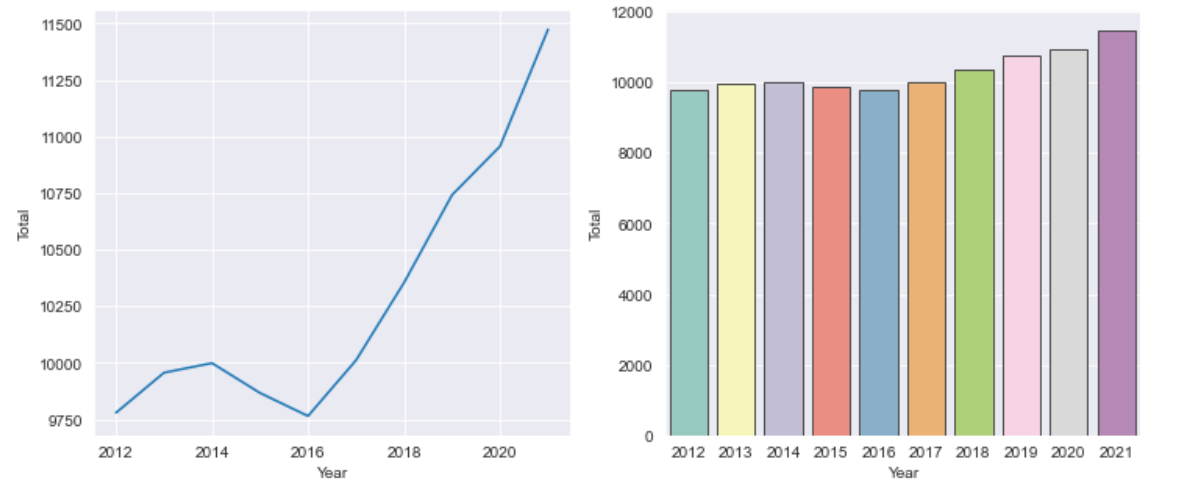## 3.中国在2012-2021年中，各种不同物质的排放趋势

``````1.#中国过去10年所有类型的物质排放趋势
2.columns = ['Coal', 'Oil', 'Gas','Cement', 'Flaring', 'Other']
3.plt.figure(figsize=(12,5))
4.fig = px.line(y=china_past_10['Total'],x=china_past_10['Year'],labels={'x':'Year','y':'Emissions'})
5.for i in columns:
7.fig.show()  ``````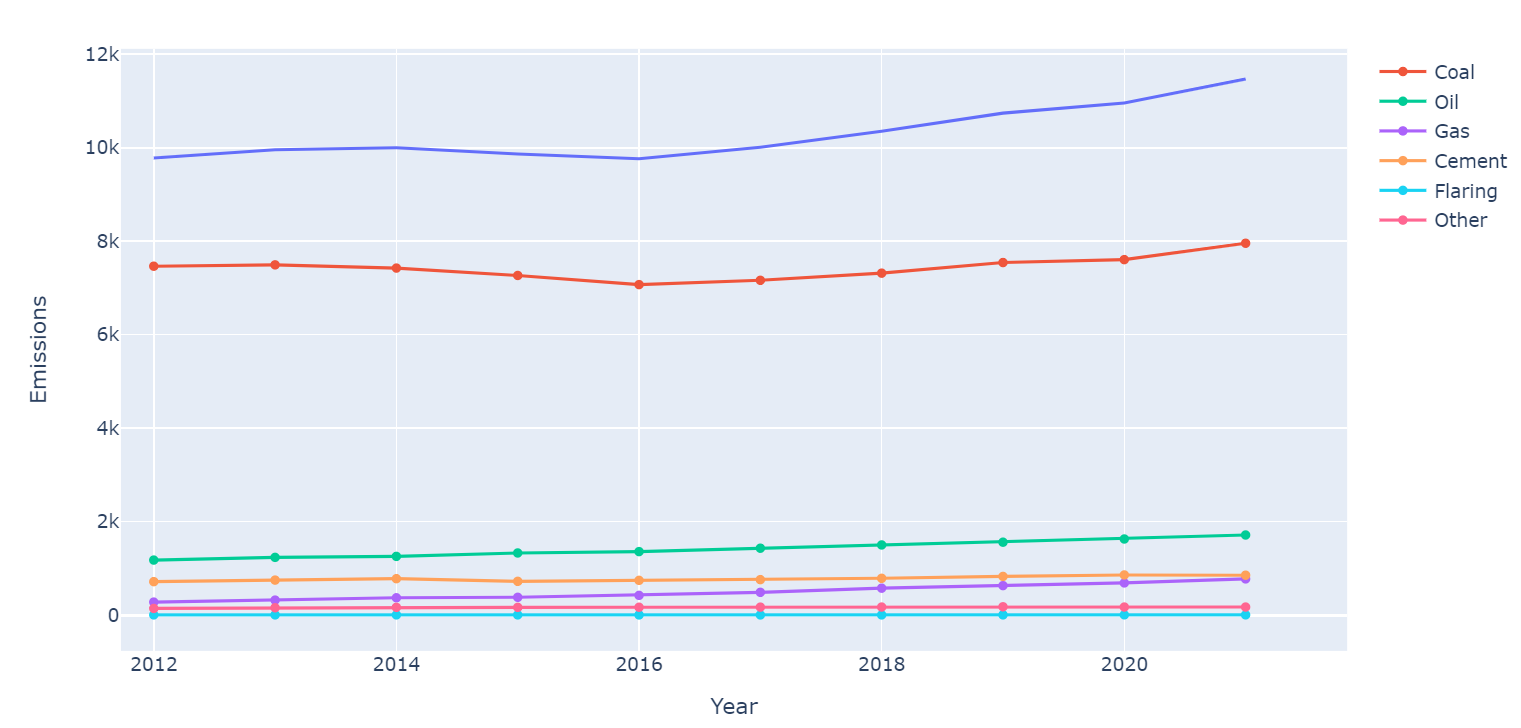## 4.各个字段的相关系数的可视化

``````1.# 相关系数分析
2.plt.figure(figsize=(12,6))
3.sns.heatmap(df.corr(),cmap='YlGnBu',annot=True)
4.plt.yticks(rotation='360')
5.plt.show()  ``````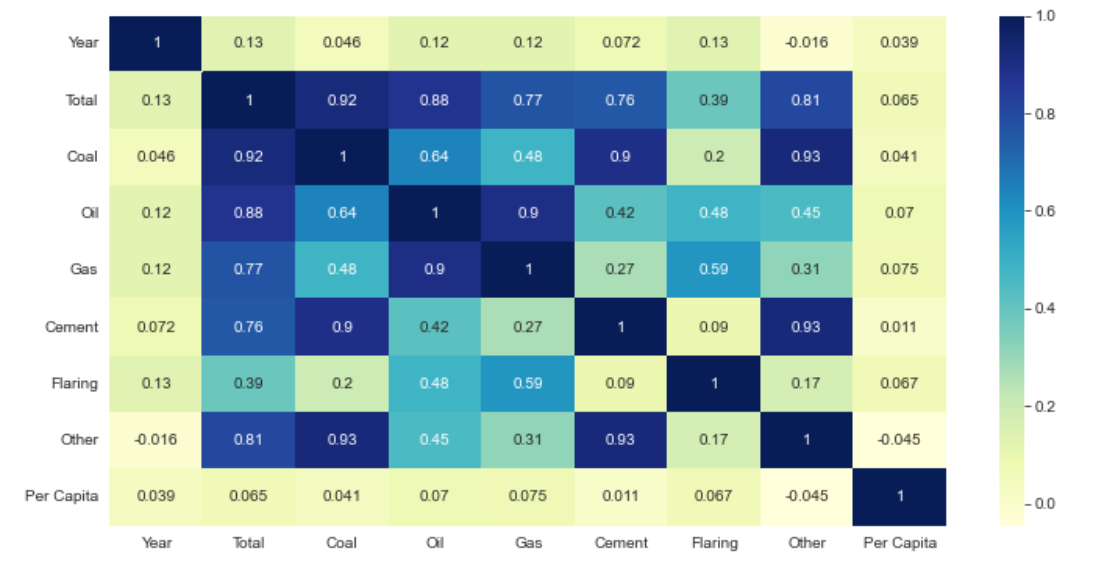## 5. 在2012-2021年中，世界和中国排放增长最快的类型

``````1.# 过去10年中，排放量增加的最快的类型
2.plt.figure(figsize=(12,5))
3.world_data_past_10 = world_data[-10:]
4.values_world = []
5.for i in columns:
6.    values_world.append(world_data_past_10.iloc[str(i)]-world_data_past_10.iloc[str(i)])
7.values_india = []
8.for i in columns:
9.    values_india.append(china_past_10.iloc[str(i)]-china_past_10.iloc[str(i)])
10.plt.subplot(121)
11.sns.barplot(x=columns,y=values_world,palette='Set2',edgecolor='.3')
12.plt.title('World')
13.plt.subplot(122)
14.sns.barplot(x=columns,y=values_india,palette='Set2',edgecolor='.3')
15.plt.title('China')  ``````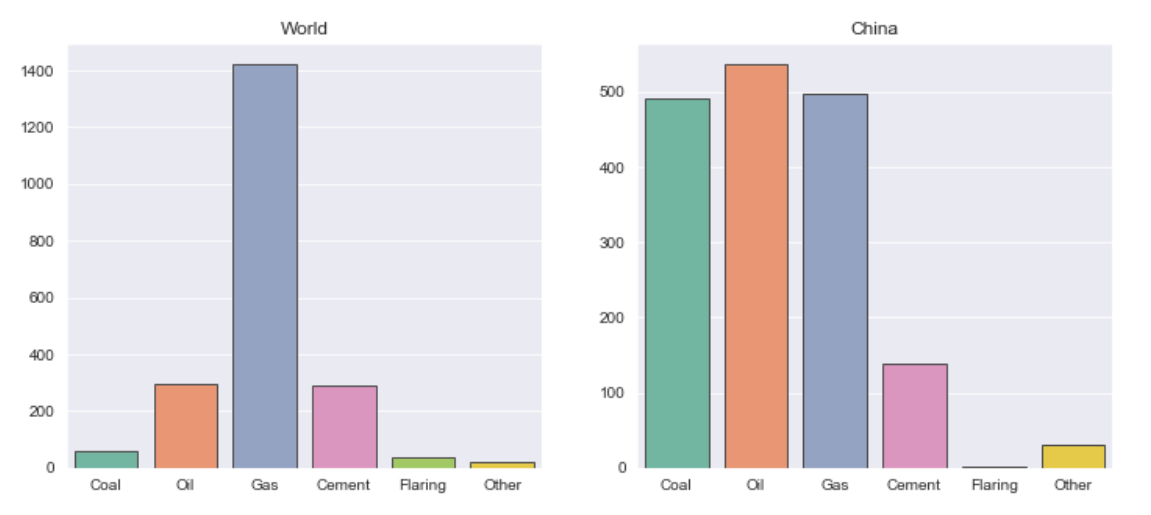## 6. 历史人均排放趋势

``````1.# 历年人均排放量趋势图
2.Year_mean_trend_pc=df.groupby(['Year'])['Per Capita'].mean().reset_index()
3.Year_mean_trend_total=df.groupby(['Year'])['Total'].mean().reset_index()
4.fig15 = px.line(Year_mean_trend_pc, x="Year", y="Per Capita")
5.fig15.update_xaxes(rangeslider_visible=True)
6.fig15.show() ``````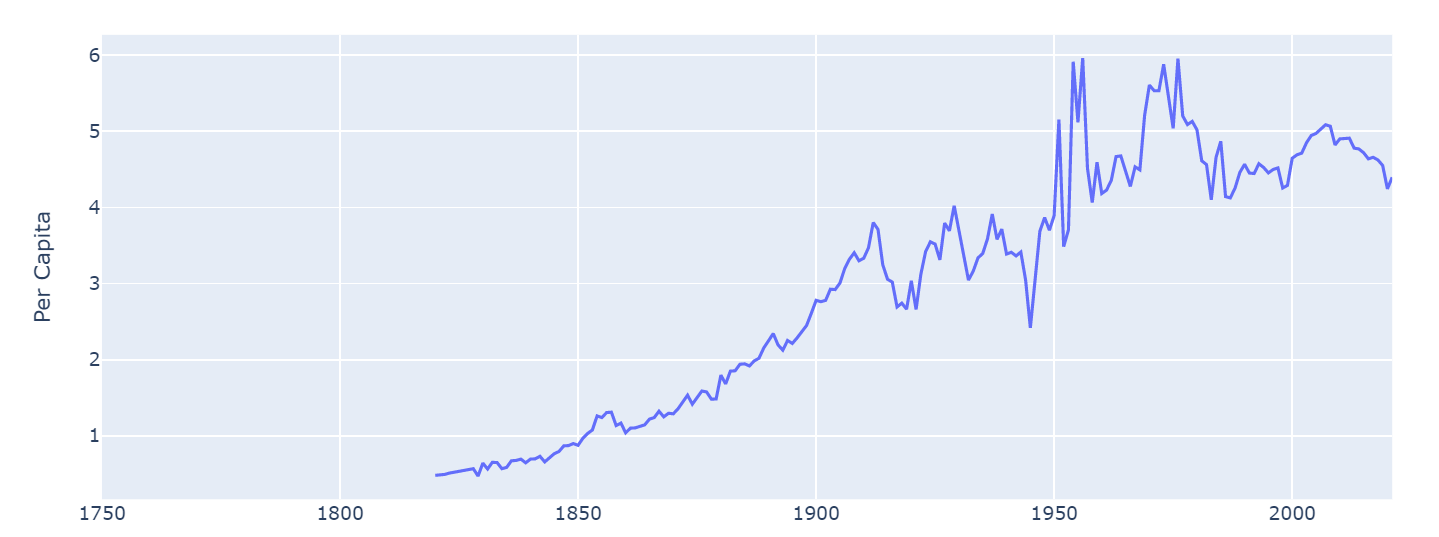## 7. 全球排放趋势折线图

``````1.years = data['Year'].unique()
2.categories = ['Coal', 'Oil', 'Gas', 'Cement', 'Flaring', 'Other']
3.plt.figure(figsize=(10, 6))
4.for category in categories:
5.    category_data = data.groupby('Year')[category].sum()
6.    plt.plot(years, category_data, label=category)
7.plt.title('Global CO2 Emission Trends by Category')
8.plt.xlabel('Year')
9.plt.ylabel('Emission (kt)')
10.plt.legend()
11.plt.show()  ``````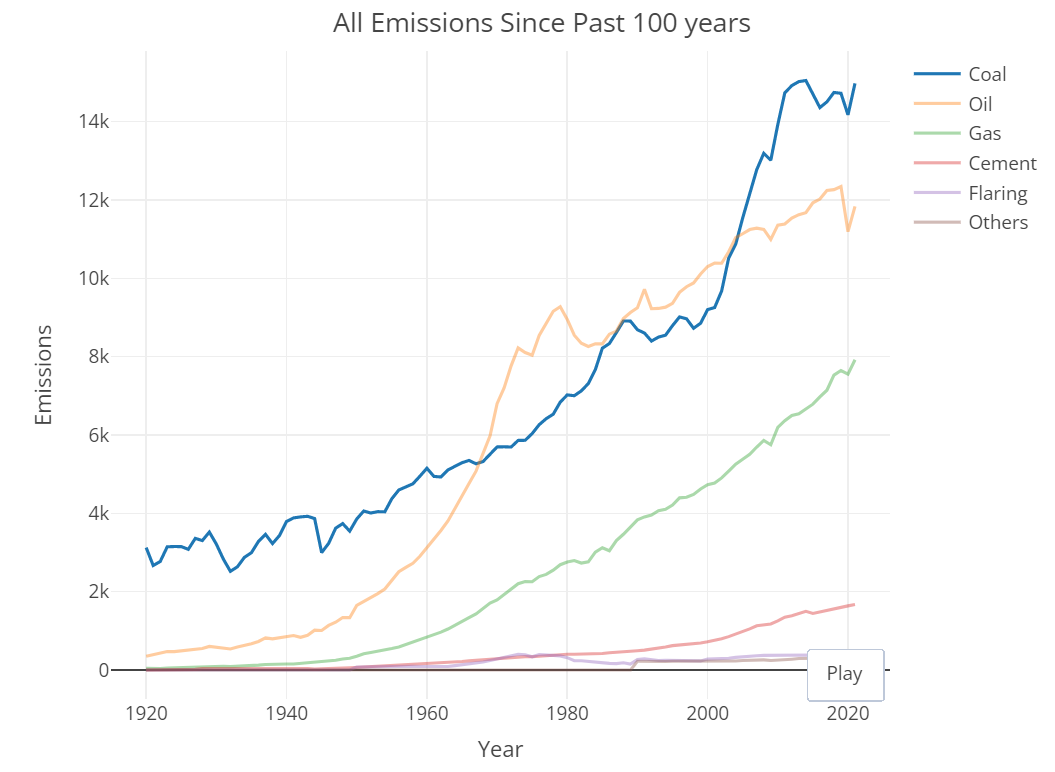## 8. 2011-2021年平均排放量最高的前10个国家

``````1.# 过去10年平均排放量最高的国家
2.plt.figure(figsize=(10,5))
3.data_past_10 = df[df['Year']>=2011]
4.avg_emissions = data_past_10.groupby('Country').mean().sort_values(by='Total',ascending=False)[:10].reset_index()
5.sns.scatterplot(x='Total',y='Per Capita',data=avg_emissions,hue='Country')``````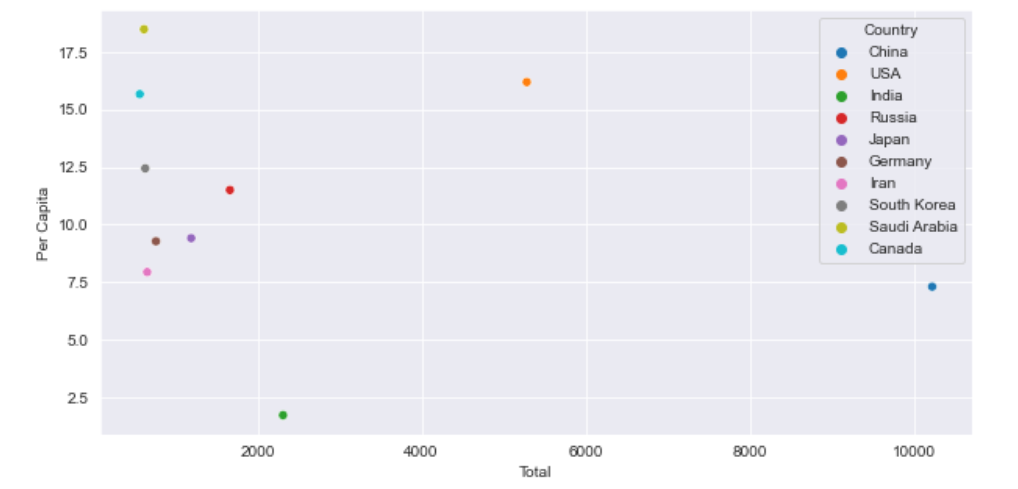## 9. 世界地图展示

``````1.# 世界地图展示
2.avg_data = data_past_10.groupby('Country').mean().reset_index()
3.countries = avg_data['Country'].values
4.ISO = []
5.cols = ['Total']+columns
6.for i in countries:
7.    ISO.append(data_past_10[data_past_10['Country']==str(i)]['ISO 3166-1 alpha-3'].unique())
8.cols.append('Per Capita')
9.colors = ['magenta','gnbu','purp','turbo','ice','curl','oxy','haline']
10.for index,i in enumerate(cols):
11.    data = dict(type='choropleth',
12.                colorscale = str(colors[index]),
13.                locations = ISO,
14.                z = avg_data[str(i)])
15.    if i!='Per Capita':
16.        layout = dict(title = str(i)+ ' Emissions By Country',
17.                      geo = dict( projection = {'type':'robinson'},
18.                                 showlakes = False))
19.    else:
20.        layout = dict(title = 'Per Capita By Country',
21.                      geo = dict( projection = {'type':'robinson'},
22.                                 showlakes = False))
23.    x = pg.Figure(data = [data],
24.                  layout = layout)
25.    po.iplot(x)  ``````